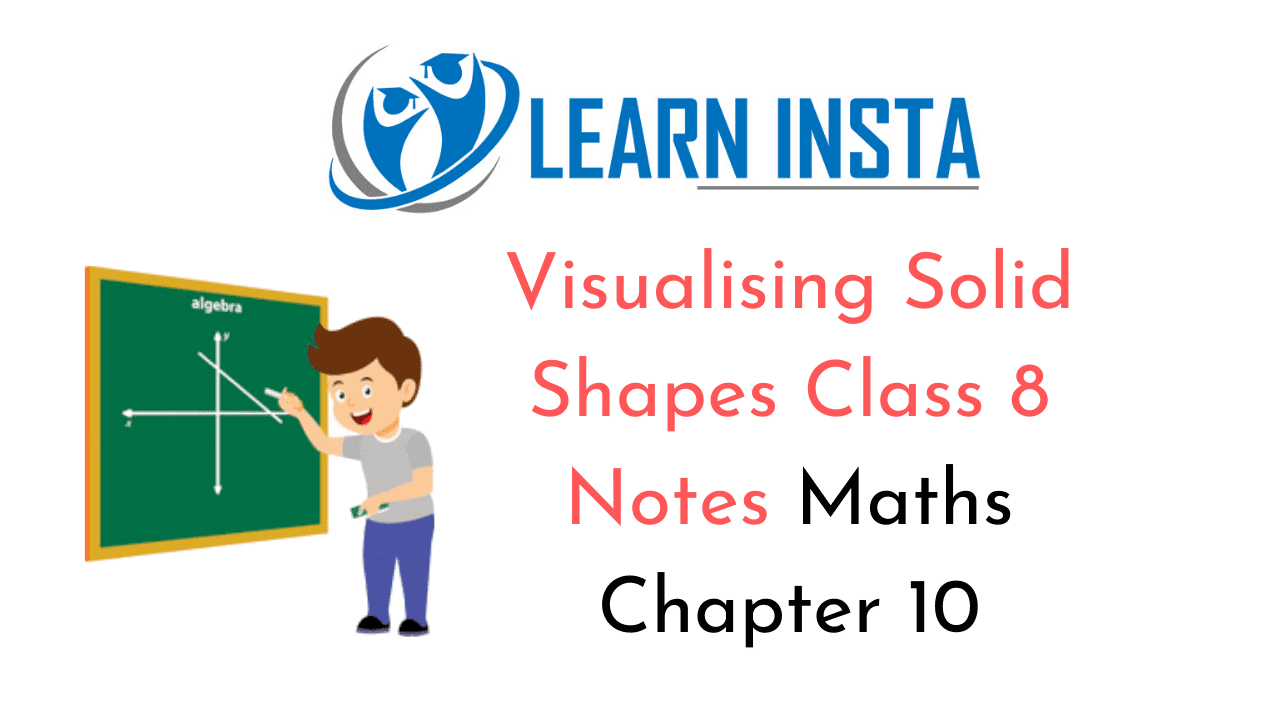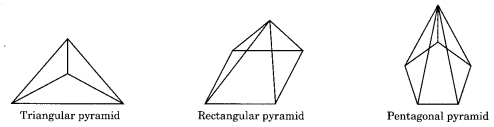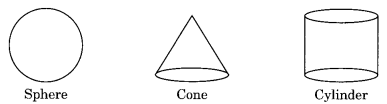On this page, you will find Visualising Solid Shapes Class 8 Notes Maths Chapter 10 Pdf free download. CBSE NCERT Class 8 Maths Notes Chapter 10 Visualising Solid Shapes will seemingly help them to revise the important concepts in less time.

## CBSE Class 8 Maths Chapter 10 Notes Visualising Solid Shapes

### Visualising Solid Shapes Class 8 Notes Conceptual Facts

3-D figures: Any shape which occupies space and has three dimensions, i.e., length, breadth and heights is called 3-D solid or a figure.

Parts of a solid objects:

• Face
• Vertex
• Edge

Types of Solids:

(i) Prism:(ii) Pyramids:(iii) Other SolidEuler’s Formula:
Number of faces + Number of vertices – Number of edges = 2